## Friday, June 16, 2006

### exam time is ahead of us!!!!!!

Today the official exams start!!! our exam is this morning and i'm so freaked out!!!!! i wish everybody luck one this exam and the rest thats going to happen the rest of this entirely really long week ahead of us so good luck everybody. this is going to be a really really long week!!!!!!

## Wednesday, June 07, 2006

### 4th growing post

Question 1: Formulas. We've learned how to write algebraic equations in the form of: mx + b = y. Convert these two sentences into algebraic equations using the above formula.

Sentence 1 :
Scott is 2 years older than Donald who turns 12 on June 21st 2006. Write this sentence in the format of mx + b = y, then convert it into an expression which allows us to figure out the age of Scott.

2 + b = y
2 + 11 = y
2 + 11 = 13

Sentence 2: Elizabeth has \$200 in her savings account. She makes \$40 every two weeks babysitting for her next door neighbours. If Elizabeth saves all of her money solve for how much money she will have in one year.

40 +200(26)=5240

Question 2: Creation: You need to create two questions for your classmates that cover different concepts (ex: T-Charts, patterns, equations, graphs etc.) that you have learned in this unit on Algebra. You then need to show how to solve the questions. Your mark will be based upon the level of question’s difficulty, and the effort put into your answer.

I'll do this tomorrow because i dont know what to put !

Question 3: Reflection: You need to look back at the chart that we filled out during the first day of the unit. This is the chart where you coloured a topic red, yellow or green. You now need to pick one concept that you coloured yellow or red and reflect in words what new skill/idea that you have learned on this topic.

Answer : Same for this one !

Question 4: Preparing for the final exam: You need to think about the year that has past in mathematics and decide which topic is your weakest, and what you need to learn during class review in order to prepare yourself for the final exam. It is not enough to say fractions, instead pick your weakest area of fractions, say the subtraction of fractions, and give an example of what you don’t understand.

Answer : And same for this one !

I'll do this tomorrow !

### Growing Post # 4

Question 1: Formulas. We've learned how to write algebraic equations in the form of mx + b = y
Convert these two sentences into algebraic using the above formula.

m: change or coefficient
b: constant or start
y: solution

Sentence 1: Scott is 2 years older than Donald who turns 12 on June 21st 2006. Write this sentence in the format of mx + b = y, then convert it into an expression which allows us to figure out the age of Scott. Scott is 13 years old and Donald will be 12 years old.

2 + b = y

2 + 11 = y

2 + 11 = 13

Sentence 2: Elizabeth has \$200 in her savings account. She makes \$40 every two weeks babysitting for her next door neighbours. If Elizabeth saves all of her money solve for how much money she will have in one year? Elizabeth will have a total of \$1160 in one year.

\$40n + \$200 = y

\$40(24) + \$200 = \$1160

Question 2: Creation. You need to create two questions for your classmates that cover different concepts (ex: T-charts, patterns, equations, graphs etc.) that you have learned in this unit on Algebra. You then need to show how to solve the questions. Your mark will be based upon the lvl of question's difficulty, and the effort put into your answer.

Bobby has \$500 dollars in his bank account and he earns \$50 every month. His friend John has \$250 in his bank account and he earns \$75 every month. How much will Bobby and John have in their bank account in one year and who will have the most amount of money? Bobby will have a total of \$1100 and John will have a total of \$1150 and John will have \$50 more than Bobby.

\$50x + \$500 = y

\$50(12) + \$500 = \$1100

\$75x + \$250 = y

\$75(12) + \$250 = \$1150

One day, Sandy and Lina decided to save money and buy a walkman for \$150 and share it with each other since they were sisters. Sandy had \$60 in her bank account and she would add \$10 everyday for 10 days while Lina had \$120 in her bank account but she would spend \$10 everyday for herself for 10 days. On the 10th day, will Lina and Sandy have enough money to buy the \$150 walkman and have leftover money? Or will they have to save more to buy a walkman? Sandy and Lina will have enough money in total to buy the \$150 walkman and have \$40 leftover.

Sandy: 10x + 60 = y

10(11) + 60 = 170

Lina: -10x + 120 = y

-10(10) + 120 = 20

Question 3: Reflection. You need to look back at the chart that we filled out during the first day of the unit. This is the chart where you coloured a topic red, yellow or green. You now need to pick one concept that you coloured yellow or red and reflect in words what new skill/idea that you have learned on this topic.

Can I use my words to create an algebraic formula?

I coloured this in red because I didn't know how to create a algebraic formula. So if I didn't know how to make one, I would probably not be able to make one from a T-Chart or use an algebraic formula to solve for an unknown. It was easier to make a formula when Mr.Reece had taught us how and it was helping me solve an unknown and make a formula by using a T-Chart.

Question 4: Preparing for the final exam. You need to think about the year that has past in mathematics and decide which topic is your weakest, and what you need to learn duriong class review in order to prepare yourself for the final exam. It is not enough to say fractions, instead pick your weakest area of fractions, say the subtraction or fractions, and give an example of what you don't understand.

My weakest topic is probably algebra. In algebra, there are always some questions that I can't figure out how to solve the unknown. But as Mr.Reece is teaching us, I am beginning to find it more easy little at a time and will be able to solve it. I also know that when the higher the grade i'm in, the harder the level of mathematics are and the harder the questions in algebra. (eaxample: -50x + 2o6 = y)

## Tuesday, June 06, 2006

### Late growing post #2

Question#1 :

(A)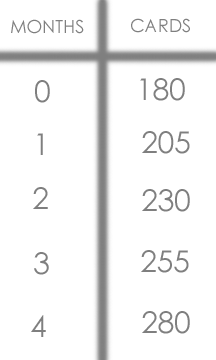(B)180 + 25 (c) = how many cards

(C) Blaank

Question #2 :

(A) If Jackie thinks there will be 4 people at the party she should buy two pizzas .

(B) If two more people show up at the party Jackie will need to order 1 more pizza.

Question #3:

(A)20+15(12)=200 Kathy will have \$200.00 in her savings account in one year.

(B)25+15(12)=205 Kathy would have \$205 in her savings account in one year.

Question#4:

BLAAANK

### LATE growing post #1

Question #1:
Rotation Symmetry means if you can rotate (or turn) a figure around a center point by fewer than 360° and the figure/shape never changes.
Reflection Symmetry is a mirrored shape. It is easy to reconize, because one half of a shape or figure is the reflection of the other half.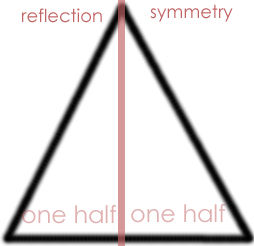Question #2: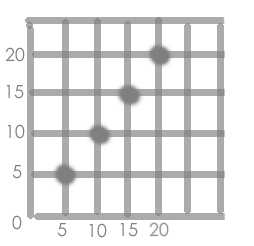Question #3:

My pattern starts with five dollars and it increases by five dollars more each step. My pattern can go throughout many steps.

Question#4: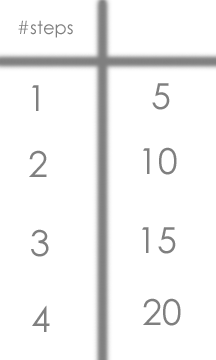^^^ \$money

## Monday, June 05, 2006

### Scibe Post For June 5, 2006

In class today we looked through some of our worksheets in our duotang and answered the questions. We answered the questions because on the test, there are some similar questions.

Page 28:
18) Using the equation 2x+1=y, create a table of values where x equals 2, 3, 5, 7, and 8.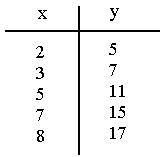19)Complete the table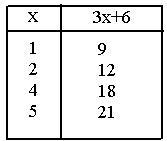Page 32:
8)What does n equal in the diagram below?
4 diamonds(n)=32
(n)=32/4 n=8

9)What does n equal in the diagram below?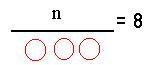n/3=8x3
x3
n=24

Page 35:
2)Six friends are going to equally share a sum of money. Each friend will receive \$9.76. Write an equation which shows the original sum of money.
n=(9.76)x6

3)Write an equation to show "sixty by a number equals three".
60/n=3

4)When a number is added to twelve, the sum is seventeen. Write this equation.
12+n=17

5)Express 4n=14
Four multipled by a number equals 14.

Page 25:
6)Using the table above, when did the temperature rise the most?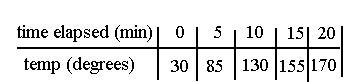85-30=55 According to the table, the temperature rose the most between 0 to 5 minutes.
130-85=45
155-130=25
170-155=15

7)Predict the temperature of the oven at the 12 minute mark.
25/5=5 degrees celcius 130+5+5=140

8)What algebraic expression would explain the relationship between Bob's and Doug's pens?
B=Bob's pens D=Doug's pens
B=2D-2 B/2+1=D

9)a)How many pens would Doug have if Bob had 16 pens.
16/2+1=9
b)If Doug had 10 pens, how many would Bob have?
2(10)-2=18

Remember that the rule has to work out with all the possibilities or else it won't work.

Lastly, we watched a brain-pop video on solving equations.

## Friday, June 02, 2006

### other half of my scribe post!!!xD

Some review from yesterday might occur in this scribe post...

Definitoins you might want to know for the exam!
Algebraic Equations

4 n + 3 = 11

An algebraic equation contains four elements : constant, coefficient,
variable and solution.

( starting point, change, variable and solution)

Start + change(variable) = solution

m x + b = y
m : coefficient
x : variable
b : constant
y : solution
Ryan purcahsed 2 CDs. one of the CDs cost \$10.00. In total they cost \$25.00.
\$10.00 + n = \$25.00
-\$10.00 -\$10.00
CD#1 = \$10.00
CD#2 = \$15.00
Always figure out the starting point first!
Homework:
You have to create a word problem and solve it.
Solving Equations
To solve on equation we need to have all variables on one side and
the constants on the otherside.
We can have only one instance of the variable.
Follow the steps to solving equations:
Step #1: Do anything in brackets.
( 7 -2 ) a = 5
5 a = 5
Step #2: combine "like" terms.
3 n + 4 n = 14
7 n = 14
Step #3: All variable on one side constants on the other.
4 m = m +3
Step #4: divide/ multiply
( 9 + 3 ) n + 6 = 8 n + 10 + 4
step #1: 12 n + 6 = 8 n + 10 + 4
step #2: 12 n + 6 = 8 n + 14
step #3: 4 n = 8 12 n - 8 n = 14 - 6
step #4: 4 n = 8
4 4
n = 2
You will nto have to do more than 2 steps on the test/exam!
T- Charts
T- charts have two columns. The second column ( y value ) is
dependant on the first column (x value )
m x + b = y
m: change or coefficient
b: constant or start
y: solution
Here is a question you could do improve your knowledge on t - charts.
You are going to the fair. It costs \$3.00 to enter
and \$1.00 per ride.
We can use the t - chart to figure out any y value if we know the change
between each step.
Graphs
We use m x + b = y
Create our graph.
• x - axis is our variable
• y - axis is our solution

Solve this next one yourself.

You are going to the fair. It costs
\$3.00 to enter and \$1.00 per ride.
If you change is increasing your graph will go up. If your change is decreasing your graph will go down.
I hope this scrbe post is some help to you! Im now going to study for exams go luck everbody!
Oh and the next scribe is.... michelle!